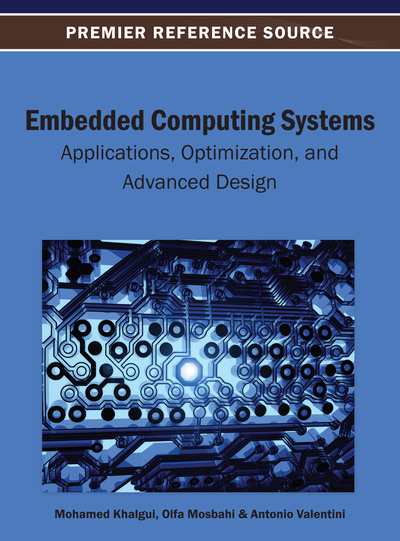# Formal Reliability Analysis of Embedded Computing Systems

Osman Hasan (National University of Science and Technology, Pakistan) and Sofiène Tahar (Concordia University, Canada)
DOI: 10.4018/978-1-4666-3922-5.ch002

## Abstract

The accurate reliability assessment of embedded systems has become a concern of overwhelming importance with their increasingly ubiquitous usage in safety-critical domains like transportation, medicine, and nuclear power plants. Traditional reliability analysis approaches of testing and simulation cannot guarantee accurate result and thus there is a growing trend towards developing precise mathematical models of embedded systems and to use formal verification methods to assess their reliability. This chapter is mainly focused towards this emerging trend as it presents a formal approach for the reliability assessment of embedded computing systems using a higher-order-logic theorem prover (HOL). Besides providing the formal probability theory based fundamentals of this recently proposed technique, the chapter outlines a generic reliability analysis methodology for embedded systems as well. For illustration purposes, two case studies have been considered, i.e., analyzing the reparability conditions for a reconfigurable memory array in the presence of stuck-at and coupling faults and assessing the reliability of combinational logic based digital circuits.
Chapter Preview
Top

## Introduction

Reliability analysis involves the usage of probabilistic techniques for the prediction of reliability related parameters, such as a system's resistance to failure and its ability to perform a required function under some given conditions. This information is in turn utilized to design more reliable and secure systems. The reliability analysis of embedded computing systems has been conducted since their early introduction. However, the ability to efficiently analyze the reliability of embedded systems has become very challenging nowadays because of their growing sizes and the complex nature of hardware software interaction.

Traditionally, simulation has been the most commonly used computer based reliability analysis technique for embedded systems. Most simulation based reliability analysis software provide a programming environment for defining functions that approximate random variables for probability distributions. The environmental behavior and the input patterns of embedded systems are random quantities and are thus modeled by these functions and the system is analyzed using computer simulation techniques, such as the Monte Carlo Method, where the main idea is to approximately answer a query on a probability distribution by analyzing a large number of samples. Statistical quantities, such as expectation and variance, may then be calculated, based on the data collected during the sampling process, using their mathematical relations in a computer. Due to the inherent nature of simulation coupled with the usage of computer arithmetic, the reliability analysis results attained by the simulation approach can never be termed as 100% accurate.

The accuracy of reliability analysis results has become imperative these days because of the extensive usage of embedded systems in safety critical areas. Some examples of safety-critical embedded systems include aircraft flight control systems, surgical robotics and patient monitoring system used in hospitals and instrumentation and control systems found in nuclear power plants. Erroneous reliability analysis in these kinds of areas could have serious consequences, such as loss of human lives.

Formal methods are capable of conducting precise system analysis and thus overcome the above mentioned limitations of simulation (Hall, 2007). The main principle behind formal analysis of a system is to construct a computer based mathematical model of the given system and formally verify, within a computer, that this model meets rigorous specifications of intended behavior. Two of the most commonly used formal verification methods are model checking (Baier, 2008) and higher-order-logic theorem proving (Harrison, 2009). Model checking is an automatic verification approach for systems that can be expressed as a finite-state machine. Higher-order-logic theorem proving, on the other hand, is an interactive verification approach that allows us to mathematically reason about system properties by representing the behavior of a system in higher-order logic.

## Complete Chapter List

Search this Book:
Reset